# Assignments

The following assignments are about solving linear equations.

1. Solve: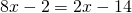Solution

2. Solve: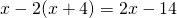Solution

3. Solve: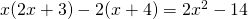Solution

4. Solve: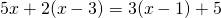Solution

5. Solve: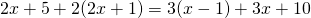Solution

6. Solve: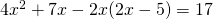Solution

7. Solve: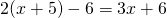Solution

8. For which value(s) of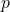does the following equation have no solution: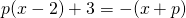Solution

9. For which value(s) of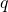is any value ofa solution of the equation: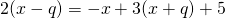Solution

10. For which value(s) ofdoes the following equation have exactly one solution: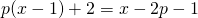Solution

0